1. The dimensional formula of E is :

(A) [MLT-2A-1]
(B) [MLT-3A-1]
(C) [MLT-2A-2]
(D) None of these

2. Which of the following has the dimensions of current ?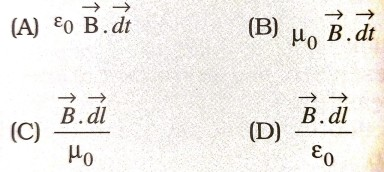3. Which of the following has the dimensions of charge ?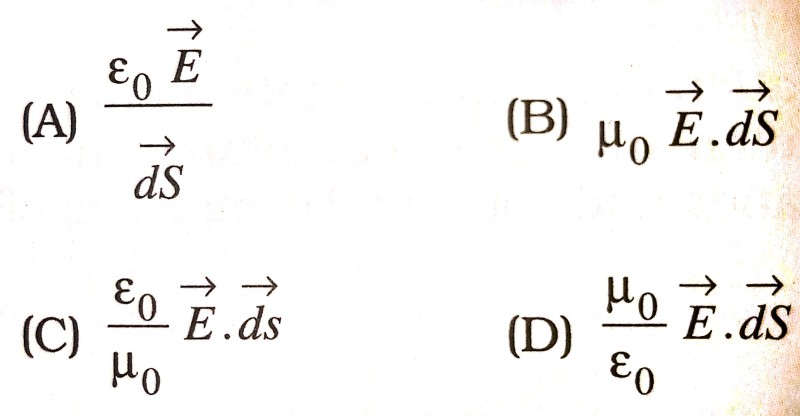4. Maxwell’s equation involving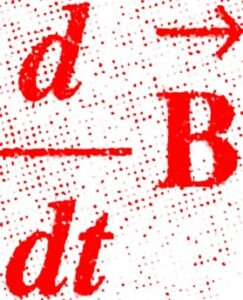is obtained from :

(A) Ampere’s law
(B) Gauss’s law
(D) Biot-Savart’s law

5. The dimensions of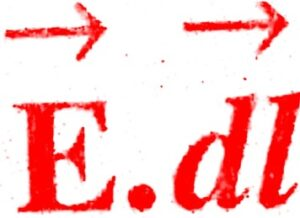are identical to that of :

(A) potential
(B) charge
(C) current
(D) None of these

6. Which of the following laws was modified by Maxwell by introducing the displacement current ?

(A) Gauss’s law
(B) Ampere’s law
(C) Biot-Savart’s law
(D) None of these

7. Choose the wave relevant to telecommunication :

(A) ultraviolet
(B) infrared
(C) microwave
(D) visible light

8. Electromagnetic waves have a speed of –

(A) 3 x 105 km s-1
(B) 3 x 106 km s-1
(C) 3 x 107 km s-1
(D) 3 x 108 km s-1

9. Which of the following EMW has highest wavelength ?

(A) X-ray
(B) ultraviolet rays
(C) infrared rays
(D) microwaves

10. Displacement current is due to :

(A) the flow of electrons
(B) the Varying electric field
(C) the ionization of atmosphere
(D) the flow of protons

11. Displacement current is always :

(A) equal to conduction current
(B) less than conduction current
(C) greater than conduction current
(D) the sum of current due to flow of positive and negative ions

12. EMW are produced by :

(A) charge in uniform motion only
(B) at rest only
(C) accelerated or deccelerated charge only
(D) all of the above

13. Who first demonstrated the existence of em waves ?

(A) Hertz
(B) Maxwell
(C) Ampere

14. If B is the magnetic field vector and É is the electric field vector in an em wave, then which of the following relations is correct ? Here c is the speed of light :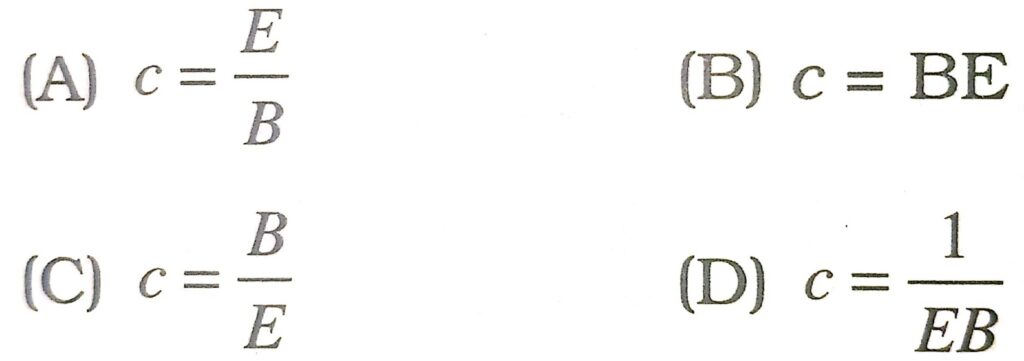15. The dimensions of E/B are same as that of :

(A) charge
(B) current
(C) velocity
(D) acceleration

16. Name the law which states that a varying electric field produces a magnetic field :

(A) Biot-Savart’s law
(C) Modified Ampere’s law
(D) None of these

17. The existence of EMW was experimentally established by :

(A) Sir S.C. Bose
(B) Maxwell
(C) Marconi
(D) Hertz

18. An EMW of v = 3 MHz passes from vacuum into dielectric medium with permittivity Er=4, then :

(A) Wavelength is doubled and the frequency remains unchanged
(B) Wavelength is doubled and the frequency become half
(C) Wavelength is halved and the frequency remains unchanged
(D) Wavelength and frequency both remain unchanged

19. Frequency of a wave is 6 x 1015 Hz. The wave is :

(B) Microwave
(C) X-ray
(D) Ultra violet

20. Choose the wrong statement ?

(A) Electromagnetic waves are transverse
(B) Electromagnetic waves travel with the speed of light in free space
(C) Electromagnetic waves are produced by accelerating charges
(D) Electromagnetic waves travel with the same speed in all media

21. If ue and um are the electric and magnetic field densities in an electromagnetic wave, then (c is the speed of light) :

(A) ue = um
(B) ue = cum
(C) ue = um/c
(D) None Of These

(A) transverse EMW
(B) longitudinal EMW
(C) both (A) and (B)
(D) None of these

23. Ampere’s circuital law was modified by :

(A) Laplace
(B) Gauss
(C) Maxwell
(D) Hertz

24. Light year is the unit of :

(A) distance
(B) time
(C) energy
(D) intensity of light

25. Which of the following helps us for recognition of precious stone ?

(A) Ultraviolet rays
(B) Infrared
(C) X-ray
(D) None

26. Oscillating electric circuits produce :

(A) Gamma rays
(B) X-rays
(C) Visible light

27. The wavelength range of visible light is :

(A) 4 x 10-7 to 8 x 10-7 m
(B) 4 x 10-6 to 8 x 10-8 m
(C) 4 x 10-5 to 8 x 10-9 m
(D) None of these

28. Choose the wave relevant to telecommunication :

(A) ultraviolet
(B) microwave
(C) infrared
(D) visible light

29. The direction of electromagnetic waves is given by :

(A) E.B
(B) ExB
(C) E/B
(D) EB

30. Velocity of light is given by :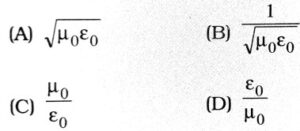31. In the above question, what is the amplitude of the oscillating magnetic field ?

(A) 1.6 x 10-3 T
(B) 1.6 x 10-6 T
(C) 1.6 x 10-3 m
(D) 1.6 x 10-7 T

32. In the above question, the total energy density (SI units) of the electromagnetic field of the wave is :

(A) 10-7
(B) 10-8
(C) 10-9
(D) 108

33. The energy density is expressed in :

(A) kg m-3
(B) Jm-2
(C) Jm-3
(D) J